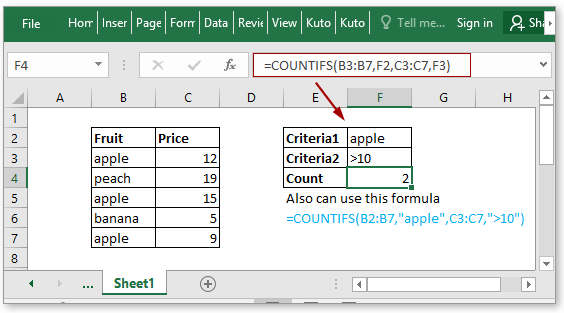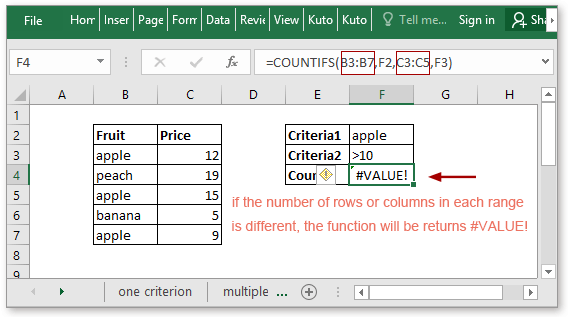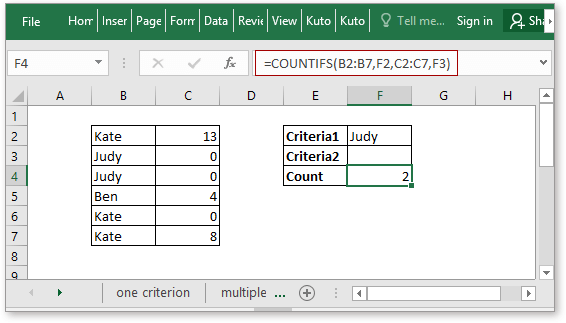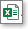Tip: Other languages are Google-Translated. You can visit the English version of this link.
Log inorRegisteror

## Excel Excel COUNTIFS function### Description

The COUNTIFS function returns the number of cells that meet one single criterion or multiple criteria. The COUNNTIFS function is one of the built-in statistical function, and it can be used as a worksheet function (WS) in Excel. The COUNTIFS function supports wildcards (? and *), and the logical values (<, >, <>, and =).

### Syntax and arguments

Formula syntax

 COUNTIFS(criteria_range1, criteria1, [criteria_range2],[criteria2],…[criteria_range_n],[criteria_n])

Arguments

 Criteria_range1: Required, the first range that you want to evaluate the first criteria. Criteria1: Required, the first criteria that define which cells will be counted. It can be in the form of number, date, text, cell reference. For example, it can be expressed as <1, A2, “KTE”. criteria_range2: Optional, the second range that you want to evaluate the second criteria. Criteria2: Optional, the second criteria that define which cells will be counted. criteria_range_n: Optional, the nth range that you want to evaluate the nth criteria, and it can be up to 127 ranges. Criteria_n: Optional, the nth criteria that define which cells will be counted, it can be up to 127 criteria.

Return Value

The COUNTIFS function returns the number of cells that meet criteria.

### Usages

Example1: Count cells based on one criterion

Supposing you want to count cells which equal to apple in column B3:B7, you can use below formula

 =COUNTIFS(B3:B7,F2)

Or

 =COUNTIFS(B2:B7,”apple”)

Press Enter key to count the number of cells that equal to apple in range B2:B7.Example2: Count rows equal to multiple criteria

If you want to count the number of apples’ prices are greater than 10, you can use below formula:

 =COUNTIFS(B3:B7,F2,C3:C7,F3)

Or

 =COUNTIFS(B3:B7,”apple”,C3:C7,”>10”)

Press Enter key to count the number of cells that equal to apple in range B2:B7 and also the prices are greater than 10.For more details about counting rows meeting multiple criteria, please go to the Count cells equal to x and y.

### Remarks

Unlike the COUNTIF function, the COUNTIFS function can apply more than one criterion, with more than one range. The criteria and ranges must be applied as pairs.

If there are more than one pair of criteria and range, the number of rows and columns of each range must be same, or the COUNTIFS function will be returns error #VALUE!.If the criteria argument is a reference to an empty cell, the COUNTIFS function will take the empty cell as zero value.If you want to find the literal question mark or asterisk mark, adding a tilde (~) in the front of the question mark (~?) or asterisk mark (~*).

### Download Sample FileClick to download COUNTIFS function sample file

#### Relative Functions:

• Excel COUNTA Function
The COUNTA function returns the number of cells that contain number, text, error value or the empty text “”, and excluding the real empty cells.

• Excel COUNTBLANK Function
The COUNTBLANK function returns the number of empty cells. The COUNTBLANK function is a built-in statistical function, which can be used as a worksheet function (WS) in Excel.

• Excel COUNTIF Function
The COUNTIF function is a statistical function in Excel which is used to count the number of cells that meet a criterion.

• Excel COUNT Function
The COUNT function is used to count the number of cells that contain numbers, or count the numbers in a list of arguments. Take an instance, COUNT(1,2,3,4,11,2,1,0) returns 8.

#### Relative Articles:

• Count cells equal to
If you want to count cells exactly match a given criterion, you can choose the COUNTIF function or the SUMPRODUCT (combine with the EXACT function) function to count cells exactly equal to without case sensitive or with case sensitive.

• Count cells not equal to
To count the number of cells that contain values that not equal to a specified value, such as below screenshot shown. In Excel, you can use the COUNTIF function to quickly handle this job.

• Count cells equal to x or y
If you want to count cells as long as that meet at least one of given conditions (OR logic) in Excel, i.e. count cells equal to either x or y, you can use several COUNTIF formulas or combine the COUNTIF formula and SUM formula to handle this job.

• Count cells equal to x and y
Here in this tutorial, it will provide some formulas to talk about counting cells that match with all given conditions, which we call it the AND logic with the COUNTIFS function.

### Excel Productivity Tools#### 300 Advanced Features Help You To Stand Out From Crowd

Would you like to complete your daily work quickly and perfectly? Kutools for Excel brings 300 powerful advanced features (Combine workbooks, sum by color, split cell contents, convert date, and so on...) and save 80% time.

• Designed for 1500 work scenarios, helps you solve 80% Excel problems.
• Reduce thousands of keyboard and mouse clicks every day, relieve your tired eyes and hands.
• Become an Excel expert in 3 minutes. No longer need to remember any painful formulas and VBA codes.
• 60-day unlimited free trial. 60-day money back guarantee. Free upgrade and support for 2 years.#### Brings Efficient Tabs to Office (include Excel), Just Like Chrome, Firefox, And New IE

• Office Tab Increases your productivity by 50% when viewing and editing multiple documents.
• Reduce hundreds of mouse clicks for you every day, say goodbye to mouse hand.
• One second to switch between dozens of open documents!Say something here...
symbols left.
###### or post as a guest, but your post won't be published automatically.
Loading comment... The comment will be refreshed after 00:00.

Be the first to comment.